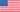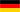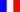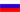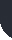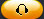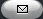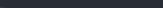Task Management Software for Team Collaboration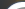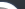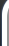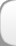HomeDownloadSupportPurchase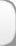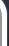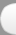Installation GuideStartUp GuideOnline Help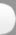# On Schedule Start Rate per Project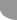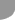Home  » Products  » VIP Task Manager Pro  » Tutorials  » Customization Guide  » On Schedule Start Rate per Project

On Schedule Start Rate per Project

On Schedule Start Rate per Project is a key performance indicator that measures the ratio of project tasks started in time relative to the number of project tasks started beyond their planned Start Date or with Start Deviation. Using the formula below you can compute On Schedule Start Rate for your projects (expressed as percentage):

On Schedule Start Rate per Project = Number of Project Tasks with Start Deviation / Number of Project Tasks Without Start Deviation.

Start Deviation is an amount of time a task falls behind the scheduled Start Date (expressed as duration).

Start Deviation = Start Date – Date Started

Start Date is a date a task is expected to start:

Date Started is a date a task is actually started.

For example, if an expected Start Date of a task is 03/12/2014 and the task has been actually started on 03/15/2012, Start Deviation is calculated as the duration between these two dates. This figure notifies that a task is 3 days or 72 hours behind the schedule.

If Start Deviation of your project tasks is greater than 0, you have a good performance in terms of Start dates of project tasks. If Start Deviation is less than 0, a task is not on schedule.

On Schedule Start Rate greater than 1 is an indicator of good performance. If On Schedule Start Rate is less than 1, it means that the bulk of your tasks is behind the schedule by Start Date.

Let’s illustrate it by an example. If total number of project tasks started in time (Tasks Without Start Deviation) is 10 and the number of tasks overdue by their Start Date (Tasks With Start Deviation) is 6, On Schedule Start Rate is calculated as
On Schedule Start Rate = 10/6 = 1.67

To set up VIP Task Manager Professional to show On Schedule Start Rate per projects please follow the instructions below:

• Add custom field “Start Deviation” with Code “cf_start_deviation”, Type “Duration” and the formula:

(\$start - \$date_started)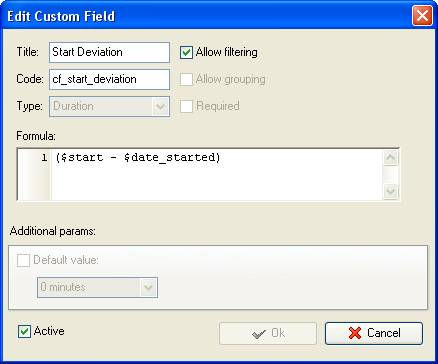• Add custom field “Tasks with Start Deviation” with Code “cf_with_start_deviation”, Type “Float” and the formula:

iif(ToString(\$cf_start_deviation) > 0, 0, iif(ToString(\$cf_start_deviation) < 0, 1,0))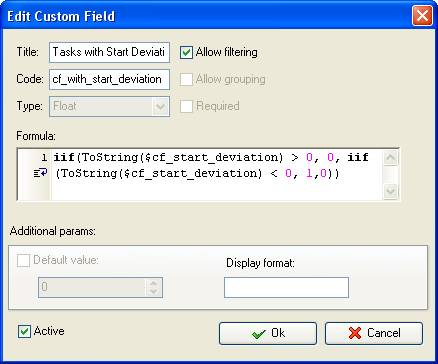If project tasks are not on track by Start date, they will be marked with the value “1” in the column “Tasks with Start Deviation”. Tasks without Start Deviation will be marked with the value “0”.

• Add custom field “Tasks Without Start Deviation” with Code “cf_without_start_deviation”, Type “Float” and the formula:

iif(ToString(\$cf_start_deviation) >0, 1, iif(ToString(\$cf_start_deviation) < 0, 0, 0))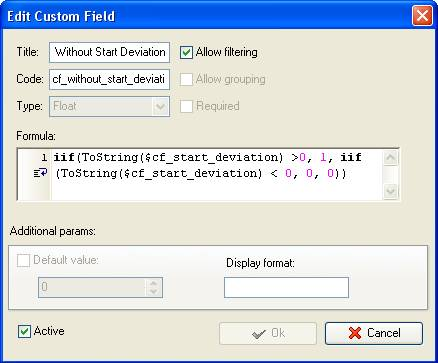The value “1” will be displayed for project tasks started in time. Tasks with Start Deviation will have the value “0” in this column.

• Add custom field “On Schedule Start Rate” with the formula:

\$cf_with_start_deviation / \$cf_without_start_deviation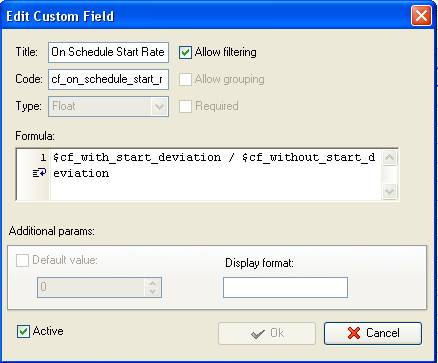• On Task Tree select project task group for which you need to display On Schedule Start Rate and select “Custom fields options”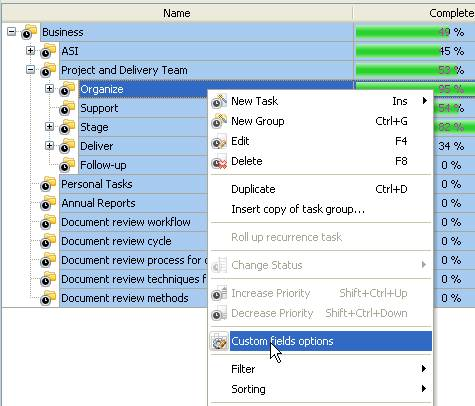• To calculate the number of tasks started in time and deviated from estimated Start Date at the task group level select project task group and set the following custom fields settings for the custom fields “Tasks With Start Deviation” and “Tasks Without Start Deviation”

- In “Settings for” select “This task group”
- select “Enable”
- in “Aggregated (for task group)” field select “Sum” from drop-down list and the corresponding custom field name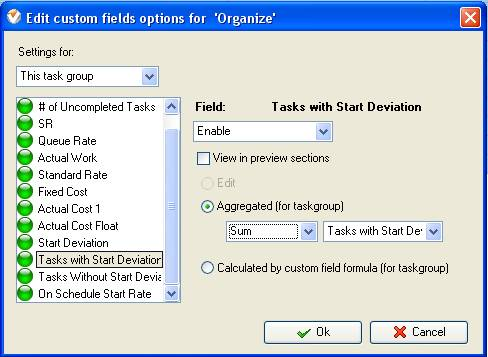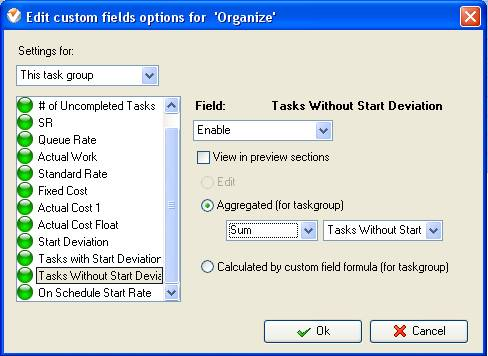• Select custom field “On Schedule Start Rate” and set the following settings

- In “Settings for” select “This task group”
- select “Enable”
- select “Calculated by custom field formula (for task group)”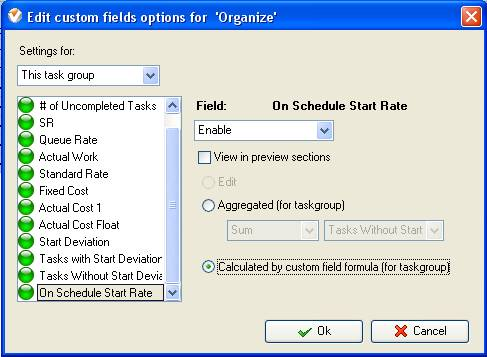If you would like to display On Schedule Start Rate for all project groups, please follow the instructions below:

• Select parent task group and set the following options for the custom fields “Tasks With Start Deviation” and “Tasks Without Start Deviation”
• In “Settings for” select “Subgroups&tasks”

- select “Enable”
- in “Aggregated (for task group)” field select “Sum”  for
For the custom field “On Schedule Start Rate” select “Calculated by custom field formula (for task group)”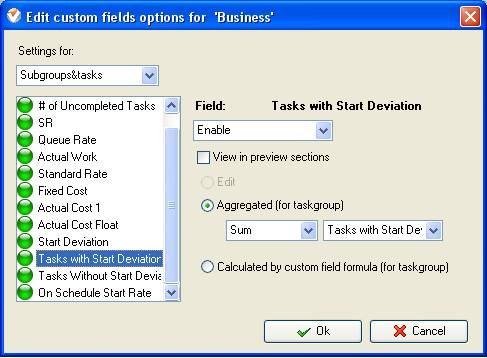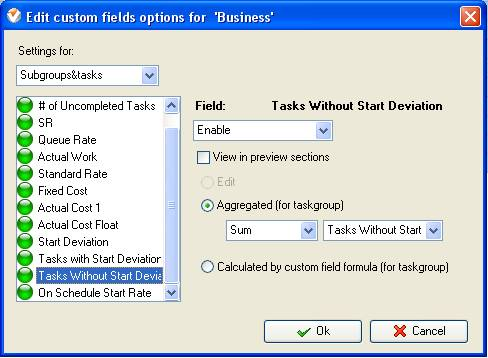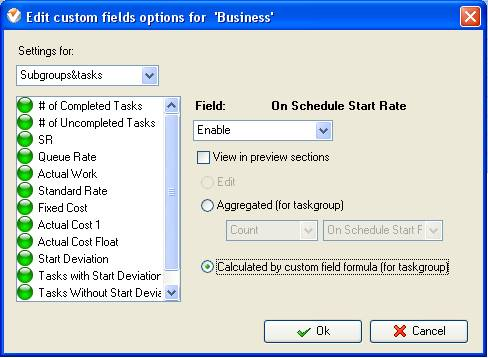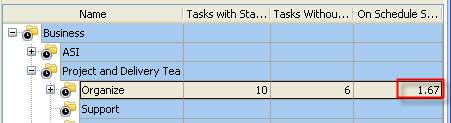On Schedule Start Rate per Project Group on Task Tree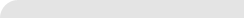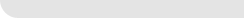» VIP Task Manager Pro VIP Task Manager Std Testimonials Turnkey Solution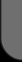ProductsSolutionsDownloadSupportPurchaseContactsForumVideoMy Account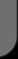Copyright © 2004 - 2023 Task Management Software